Problems & Puzzles: Collection 20thColl.20th-006. Prime squaring the Prime square.

On March 13, 2018, Vic Bold sent the following puzzle:

Fill a square of size PxP with P prime and the minimal quantity K of smaller prime squares, such that K is prime too.

Example:

For P=11, K=15 (fail!... because K is not prime)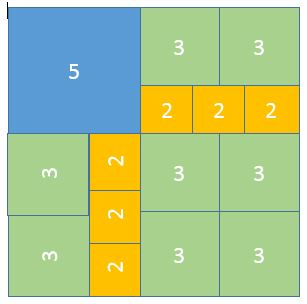Q1. Send the minimal solution with K prime.

Q2. Send a solution using K distinct prime squares.

Q3. Is possible produce solutions such that no line can cut the big square in two parts without cutting at least one small square? (In the example given above, two lines can divide completely the big square.)

Contributions came from Fred Schneider, Giovanni Resta, Jan van Delden and Emmanuel Vantieghem.

***

Fred wrote on June 30, 2018: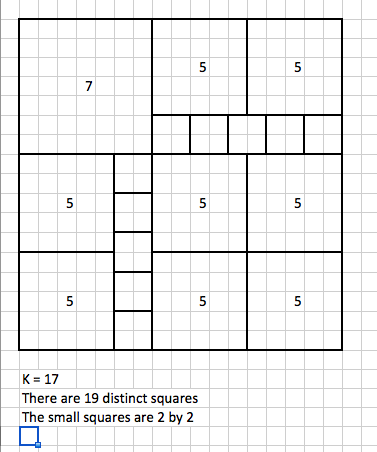***

Giovanni wrote on July 1, 2018:

The minimal solution to both Q1 and Q3 is the attached dissection
square13.png, A 13x13 square into two 5x5, seven 3x3, and fourteen
2x2 for a total of 2+7+14=23 squares.

You may also like the smallest square with prime side that can be
dissected into a prime number of squares with prime sides, and such
that each size appears a prime number of times. It is the attached
square37.png.

A square with side 37 dissected into 83 squares:
2 of side 11,  5 of side 7, 13 of side 5, 61 of side 3, and 2 of side 2.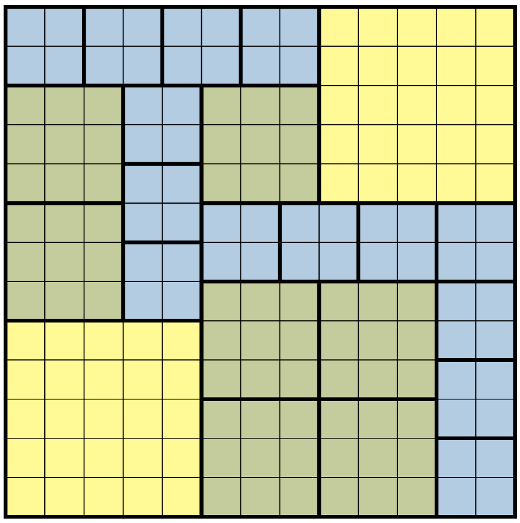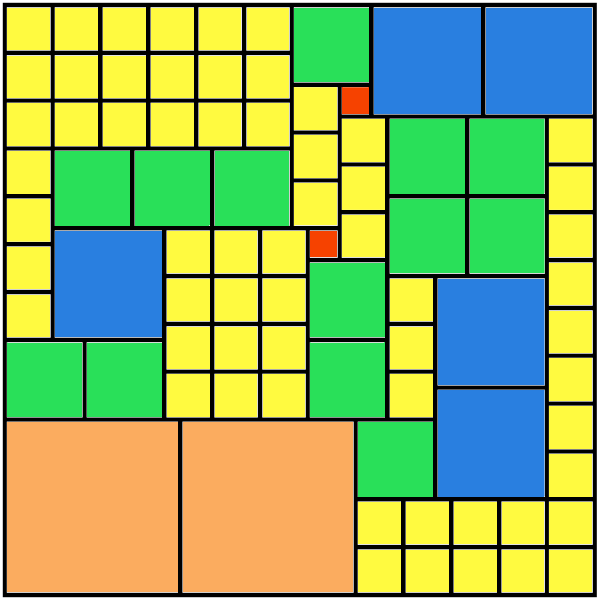Are you trying for a solution using only distinct prime squares?

No. Tiling a square (prime or not) with distinct prime squares is not
going to be easy.

At the site http://www.squaring.net there are a lot of tilings
with distinct squares and if I'm not wrong they talk about the

http://www.squaring.net/sq/ss/s-pss.html#prime-elements-pss

It seems to me they show the tiling with the largest proportion
of primes they found in their very extensive search is one which is
about 56%, that is 18 primes over 32 subsquares.

***

Jan wrote on July 2, 2018:

Define:

n: number of tiles with side 2
n: number of tiles with side 3
n[p[i]]: number of tiles with side p[i]>3

n[p]:    sum(n[p[i]])

We have:

n+n+n[p]=K

4.n+9.n+sum(n[p[i]].p[i]^2)=P^2

If one computes the last expression mod 3 or mod 4:

n+n[p]=1 mod 3
n+n[p]=1 mod 4

Where I used:  P^2,p[i]^2=1 mod 3 and P^2,p[i]^2=1 mod 4, for p[i]>3, P>3.

So this could reduce the number of computations somewhat.

Q1:

K=19, P=17And other configurations of these tiles, including symmetries.

Q2:

To find such a solution is a real challenge!

Q3:

K=23, P=13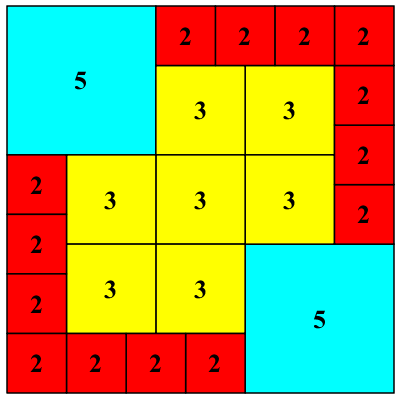And other configurations of these tiles, including symmetries.

***

Emmanuel wrote on July 7, 2018:

In attachment you find what I think is the minimal solution :
Nineteen squares filling a 17x17 square :
one square is 7x7
eigth squares are 5x5
ten squares are 2x2.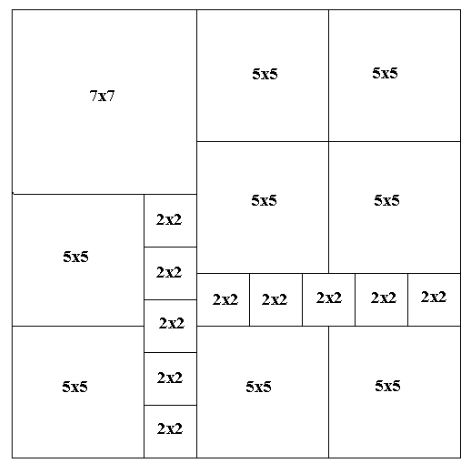For every P  between 17 and 997  I was able to find solutions (that are minimal in my opinion).
It was easy to find them such that there was only one line that does not cut any of the smaller squares
(as you can see in the example).  It was impossibe to find solutions without such lines.

***Records   |  Conjectures  |  Problems  |  Puzzles